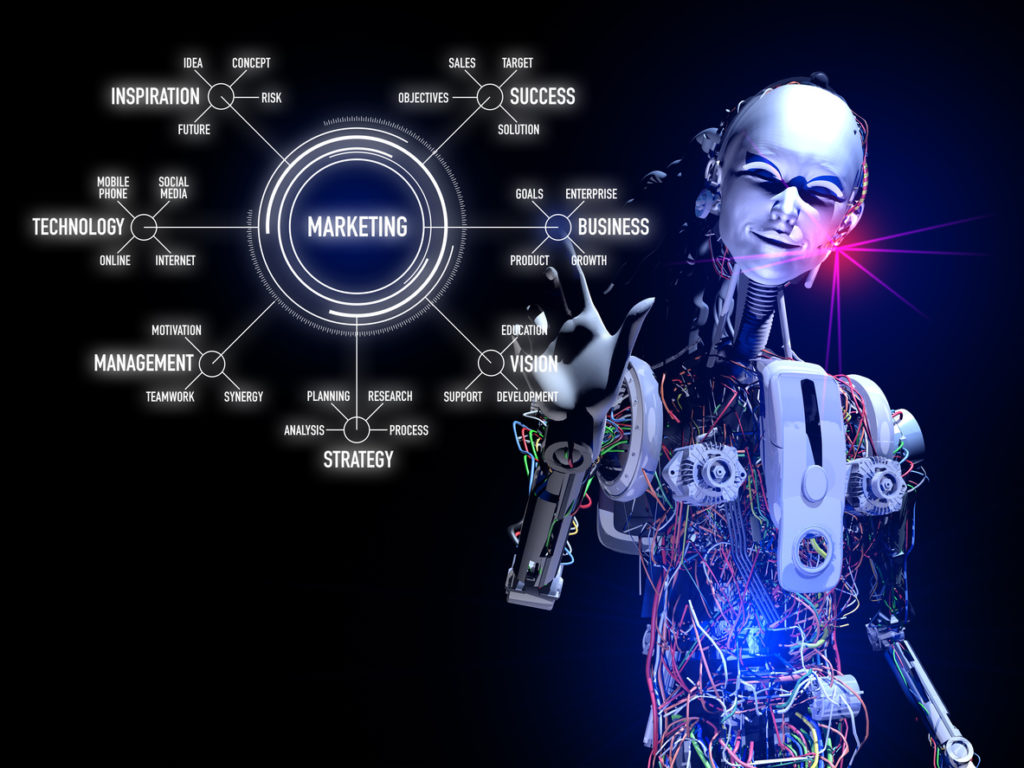## WHAT IS NEURAL NETWORK IN DEEP LEARNING ( AI ) ?

Neural networks is method through which deep learning learns structure of data. As the name suggest neural which is in biology means cells (Neuron) in our brain and network means connection of neural in such fashion which can learn representation of data and can learn dependency of each variable in data from one and other. Some of the concept may seen new to you. We will try to explain each one in detail.

As in our brain each neuron is responsible to learn some thing of data which we perceive through our five sense organ such as we see image of apple than some of the neuron in our brain learn about edges of an apple which will always give us feel that this an apple like wise each neuron is responsible to learn something of data which in future responsible to predicting correct things.

Geoffrey Hinton developed this technique and said that whenever linear function is passed through activation function than result represent a non linear function which is highly resemble with the universal approximation theorem which state that any thing in this world can be represented through function.In Neural network we accomplished Universal approximation theorem.

## HOW IT WORKS

You have to defined three things to make it work which is DATA, ARCHITECTURE and LOSS function.

## DATA

You need data to learn data can be of any form such as Image, Audio, Video, tabular data. Neural net will learn symmetry of data with the label.

## ARCHITECTURE

You need to defined architecture so that network can learn fast and effectively. Architecture means stacking and arranging different linear layers to one and other which can learn data representation fast. Some famous architecture for image recognization is VGG16, VGG19, RESET, XCEPTION, DENSENET and many more.

## LOSS FUNCTION

You need to defined some less function which can give you value that how much data is learned properly. It will match already labeled data will prediction and find it how much different is there in between your network result and actual prediction. We will try to reduce this number as much as possible. Popular loss function in RMSE ( root means square error), Categorical entropy loss function.

## DIFFERENCES WITH MACHINE LEARNING ALGO

Main difference between machine learning algorithm such as random forest, xgboost tree is that all calculated average or assembling different averages between target and prediction. They are less accurate but easy to implement. They all create decision trees and each node assign some numeric value based on target. It really create good representation of seen data. Many times fast on unseen data. Lets take an example of times series whose value is dependent on time. Random forest and xgboost tree really works good on time data which is already seen but will be very less accurate on future prediction. But neural nets understand and learn representation of time data and also predict good result on unseen time data also.

## TECHNICAL UNDERSTANDING

Neural network is method to developed software through numbers. Each function represented as a number but say you have linear equation as

### y=ax+b

Here every symbol in above equation is a number and each number is represented by matrics

In the above equation input image is hand written text which is represented with pixel value of one.

## PARAMETER

This a the learning variable which is learned through all this process. Bias is also variable which is learned through all this process.

## PREDICTIONS

This is also matrices which is absolute number predicted by above equation.Above equation is hypothetical do not calculate it. It will give wrong results. This is just explain you how linear equation is represented in deep learning.

All learnable numbers are called parameters and all learned numbers are called activation.

## REQUIREMENT

To enroll or start any deep learning course yo need to have at least higher mathematical knowledge and some experience in coding with any language. We will also covers math's topic and program language topics which is least required for deep learning course.

## CONCLUSION

In this post we have learn't about neural networks and how its work to learn from data. We have also seen importance of architecture, data and loss function.If you have any query or feedback or need any help regarding boiler code of any topic , you can freely ask in comments. I would really love to help you.